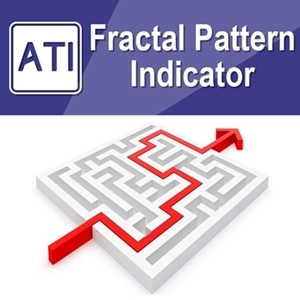•### Introduction to Fractal Pattern Indicator

Overview on Fractal Pattern Indicator

Fractal Pattern Indicator is the first indicator that allows you to analyse Fractal Patterns in price and time. Its concept and practical application were originally developed and researched by Young Ho Seo.

In Fractal space, price and time are the independent variables that we have to predict in our trading. The indicator is designed to predict the moves in  price and time. Considering that time based strategy is rare, this is very unique indicator with full range of time analysis.

In doing so, this indicator uses the Fractal Decomposition Analysis (FDA technique was originally created by Young Ho Seo). Fractal Decomposition Analysis allows us to study the seperate Fractal Cycles in order (i.e. also known as Fractal Cycle Analysis).

The indicator will output the probability information for the future turning point projection. You have an access to this probability information for small, medium, and large fractal cycles at the same time.

Main Features with Fractal Pattern Indicator

1. Automated Gann’s Angles (Gann’s Fan) – measure trend strength with angled lines

2. Detect Turning Point Probability in price dimension (i.e. amplitude) and time dimension (i.e. wavelength)

3. Fibonacci Probability Graph helping to detect more accurate rising wedge, falling wedge and triangle patterns

4. Improve trading performance with Harmonic Pattern and X3 patterns with probability filter

5. Trading with Mother Wave with high turning point chance

6. any other innovative methods

Fractal Pattern Indicator Manuals

Fractal Pattern Indicator Strategy Guides

Introduction to Fractal Pattern in Financial Market

Trading Price Pattern with Turning Point Probability

Fractal Moving Average Indicator

Every Fractal Pattern Indicator users are adviced to use it with Fractal Moving Average Indicator together. The full features of Fractal Moving Average indicator can be used with Fractal pattern Indicator. It is free.

Important Note about Fractal Pattern Indicator

Please read the provided manuals and strategy guides thoroughly before using Fractal Pattern Indicator. This is a must before using Fractal Pattern Indicator.

Further Note about Fractal Pattern Indicator

Fractal Pattern Indicator can be used on its own. However, because this indicator provides valuable probability information for your trading, you can always combine with different trading system. Following indicators can be used together with Fractal Pattern Indicator.

Published Current Version Updated Visits
18 Dec 2019 12.8 27 March 2020 1000

### More Products

PriceFreePaidPaid
Just enter 00000 to get going
in the Order ID field in